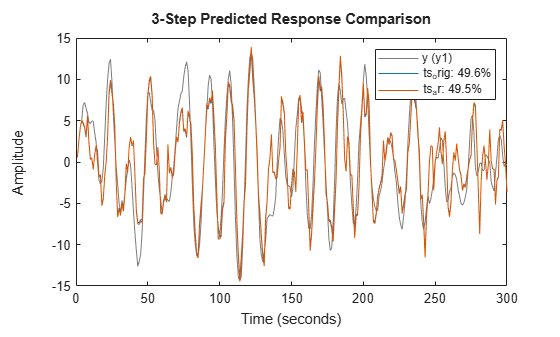Documentation

## Identify Time-Series Models at the Command Line

This example shows how to simulate a time-series model, compare the spectral estimates, estimate covariance, and predict output of the model.

Generate time-series data.

```ts0 = idpoly([1 -1.5 0.7],[]); e = idinput(200,'rgs'); y = sim(ts0,e); y = iddata(y); plot(y);````y` is an `iddata` object with sample time 1.

Estimate and plot the periodogram and power spectrum.

```per = etfe(y); speh = spa(y); spectrum(per,speh,ts0);```Estimate a second-order AR model and compare the spectrum plots.

```ts2 = ar(y,2); spectrum(speh,ts2,ts0);```Define the true covariance function.

```ir = sim(ts0,[1;zeros(24,1)]); Ry0 = conv(ir,ir(25:-1:1)); ir2 = sim(ts2,[1;zeros(24,1)]); Ry2 = conv(ir2,ir2(25:-1:1));```

Estimate the covariance.

```z = [y.y;zeros(25,1)]; j = 1:200; Ryh = zeros(25,1); for k=1:25, a = z(j,:)'*z(j+k-1,:); Ryh(k) = Ryh(k)+conj(a(:)); end Ryh = Ryh/200; % biased estimate Ryh = [Ryh(end:-1:2);Ryh];```

Alternatively, you can use the Signal Processing Toolbox™ command `xcorr`.

`Ryh = xcorr(y.y,24,'biased');`

Plot and compare the covariance.

`plot([-24:24]'*ones(1,3),[Ryh,Ry2,Ry0]);`Predict model output.

`compare(y,ts2,5);`## More About

##### SupportGet trial now